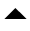# Alphanumerical Series Quiz for RRB PO & Clerk exam : Quiz 10

## Alphanumerical Series Quiz for RRB PO & Clerk exam : Quiz 10

Alphanumeric series questions are made up of a mix of numbers, alphabets, and symbols, and applicants must answer questions depending on the alphanumeric series.
The Alphanumeric series generally cover 3-5 questions in the reasoning ability section of many Government exams and is one of the most commonly asked topics.
Candidates who are studying for future competitive exams should focus on “alphanumeric series” topic in order to crack the exam on the first try and score good marks.
The Alphanumeric series questions are scoring and one of the most prevalent reasoning topics, in addition to being simple to answer.
We’ve compiled a quiz of 10 alphanumeric series questions, along with their detailed answers, in this article.

Directions (1-5): Study the following arrangement carefully and answer the questions given below:1. Which of the following element is tenth to the right of the ninth element from the left end if the first thirty elements of the above arrangement are reversed?
(a) 5
(b) 9
(c) J
(d) D
(e) None of these

Ans. b
Sol. The new series obtained after the change is as follows:-
A I @ R X W S ∆ H C D J N M # Q 6 1 9 5 L T F * ₤ U G 3 2 B 4 7 Z \$ V E Y 8
Now, the required element is (10+9) = 19th element from the left, i. e. 9.

2. What should come in place of the question mark (?) in the series given below based on the given arrangement?
BG2 *LF 1?6 JHD
(a) S
(b) N
(c) #
(d) M
(e) None of these

Ans. c
Sol. BG2 *LF 1#6 JHD

3. How many such consonants are there in the above arrangement each of which is immediately preceded by a number but not immediately followed by a symbol?
(a) None
(b) One
(c) Two
(d) Three
(e) None of these

Ans. b
Sol. We have to search for number – consonant – number sequence and number – consonant – Letter
sequence. Only ‘G’ is such a consonant.

4. Four of the following five are alike in a certain way and so form a group. Which is the one that does not belong to that group?
(a) #NDH
(b) SX@A
(c) 7\$E8
(d) G₤FL
(e) #69L

Ans. e
Sol. Others are in order from left to right

5. If every fifth character starting from U (delete U first) is deleted, then which character will be exactly midway between the tenth element from the left and the same-positioned element from the right, in the newly obtained series?
(a) J
(b) #
(c)₤
(d)(e) None of these

Ans. a
Sol. The new series obtained is as follows:-
B 2 3 G ₤ * F T 5 9 1 6 # M N J C HS X R @ I 4 7 Z \$ E Y 8
Hence, the required element is J.

Directions (6-10): Study the following arrangement of combinations for English letters, numerals and symbols carefully and answer the questions given below it:
8 C M @ N & T 2 Y 6 S & Q \$ 7 * W Z 3 U E % A 4
6. If each symbol is first converted into a numeral and then all the numerals are converted into English letters, how many such converted English letters will be there in the above arrangement of element?
(a) 25
(b) 7
(c) 12
(d) 13
(e) None of these

Ans. c
Sol. Required number of letters = (8, @, &,2,6,&, \$,7, *, 3,%, 4)= 12

7. How many such symbols are there in the above series each of which is immediately preceded and also immediately followed by vowel?
(a) One
(b) Two
(c) Three
(d) Nil
(e) None of these

Ans. a
Sol. There is only such symbol (E%A)

8. What should come in place of the questions mark (?) in the following series based on the above arrangement?
CMA N&E 2Y3 (?) \$77
(a) S6&
(b) S&Z
(c) S&W
(d) &Q#
(e) None of these

Ans. c
Sol. The series is —
CMA, N&E, 2Y3, S&W……

9. If all the vowels are dropped from the above series, which of the following would be the 8th element to the right of the 13th element from the left end?
(a) 4
(b) %
(c) 8
(d) C
(e) None of these

Ans. a
Sol. R=8th
L=13th
L=21th = 4

10. Four of the following five are alike in a certain way with respect to their position in the above arrangement. Which is one that is different from the other four?
(a) E3W
(b) Q7W
(c) &62
(d) TMN
(e) 4% U

Ans. d
Sol. Except (d), in other groups, there is a gap of one letter/symbol between two.

#### Aatma Nirbhar Reasoning Topic Wise Online Test Series

Recommended PDF’s for:

### Preparation Kit PDF

#### Most important PDF’s for Bank, SSC, Railway and Other Government Exam : Download PDF Now

AATMA-NIRBHAR Series- Static GK/Awareness Practice Ebook PDF Get PDF here
The Banking Awareness 500 MCQs E-book| Bilingual (Hindi + English) Get PDF here
AATMA-NIRBHAR Series- Banking Awareness Practice Ebook PDF Get PDF here
Computer Awareness Capsule 2.O Get PDF here
AATMA-NIRBHAR Series Quantitative Aptitude Topic-Wise PDF 2020 Get PDF here
Memory Based Puzzle E-book | 2016-19 Exams Covered Get PDF here
Caselet Data Interpretation 200 Questions Get PDF here
Puzzle & Seating Arrangement E-Book for BANK PO MAINS (Vol-1) Get PDF here
ARITHMETIC DATA INTERPRETATION 2.O E-book Get PDF here
3